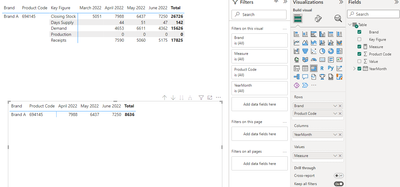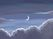cancel
Showing results for
Did you mean:

## How to calculate stocks at end of each month by using DAX?

Hi All ,

I have a scenario now and struck with the output as per below requirement :

Calculate how much stock available at End of each Month by DAX (Logic: Prev.Month Closing Stock + Cur.Month Production + Cur.Month Receipts - Cur.Month Demand)

 Brand Product Code Key Figure MAR 2022 APR 2022 MAY 2022 JUN 2022 Brand A 694145 Demand 4,653 6,611 4,362 Brand A 694145 Receipts 7,590 5,060 5,175 Brand A 694145 Production 0 0 0 Brand A 694145 Closing Stock 5,051 7,988 6,437 7,250 Brand A 694145 Days Supply 44 51 47

example : 7,988 closing stock value in April 2022 comes as per formula logic which mentioned above (5051+0+7590-4653) like this same followed for all months .
So this formula needs to be done in Power BI using DAX .

Status: Investigating

I did a simple change on your data. You may see like below：

``````let
Source = Table.FromRows(Json.Document(Binary.Decompress(Binary.FromText("dY/LDoMgEEV/hbBmAcKALNv6AU1dGhZGTUNqgfhY+PdFtF2VxWReOXfmNg2+Tq3r0QUTLLVgAmJRDe84iwWKIYgEvm+JZCz1XBbYkL/kY+gGG5b5ZBUBTWMGQuWRmYIce598v3aL9e6k6S8yxG30s3VPVC++ex1ngKWzuizTy4Kr1BeQFanabUb1GsK4fR2LXSt5VdiYDw==", BinaryEncoding.Base64), Compression.Deflate)), let _t = ((type nullable text) meta [Serialized.Text = true]) in type table [Brand = _t, #"Product Code" = _t, #"Key Figure" = _t, #"MAR 2022" = _t, #"APR 2022" = _t, #"MAY 2022" = _t, #"JUN 2022" = _t]),
#"Changed Type" = Table.TransformColumnTypes(Source,{{"Brand", type text}, {"Product Code", Int64.Type}, {"Key Figure", type text}, {"MAR 2022", Int64.Type}, {"APR 2022", Int64.Type}, {"MAY 2022", Int64.Type}, {"JUN 2022", Int64.Type}}),
#"Unpivoted Columns" = Table.UnpivotOtherColumns(#"Changed Type", {"Brand", "Product Code", "Key Figure"}, "Attribute", "Value"),
#"Changed Type1" = Table.TransformColumnTypes(#"Unpivoted Columns",{{"Attribute", type date}}),
#"Renamed Columns" = Table.RenameColumns(#"Changed Type1",{{"Attribute", "YearMonth"}})
in
#"Renamed Columns"``````

``````Measure =
var _a = CALCULATE(SUM('Table'[Value]),FILTER(ALLEXCEPT('Table','Table'[Brand],'Table'[Product Code]),[Key Figure]=	"Closing Stock"&&[YearMonth]=EDATE(MAX('Table'[YearMonth]),-1)))
var _b = CALCULATE(SUM('Table'[Value]),FILTER('Table',[Key Figure]="Production"))
var _c = CALCULATE(SUM('Table'[Value]),FILTER('Table',[Key Figure]="Receipts"))
var _d = CALCULATE(SUM('Table'[Value]),FILTER('Table',[Key Figure]="Demand"))
return _a+_b+_c-_d``````

Then you will get a result like：Best regards.
Community Support Team_ CaitlynHelper I

Can you guys pls help on this topic ?Community Support
Status changed to: Investigating

I did a simple change on your data. You may see like below：

``````let
Source = Table.FromRows(Json.Document(Binary.Decompress(Binary.FromText("dY/LDoMgEEV/hbBmAcKALNv6AU1dGhZGTUNqgfhY+PdFtF2VxWReOXfmNg2+Tq3r0QUTLLVgAmJRDe84iwWKIYgEvm+JZCz1XBbYkL/kY+gGG5b5ZBUBTWMGQuWRmYIce598v3aL9e6k6S8yxG30s3VPVC++ex1ngKWzuizTy4Kr1BeQFanabUb1GsK4fR2LXSt5VdiYDw==", BinaryEncoding.Base64), Compression.Deflate)), let _t = ((type nullable text) meta [Serialized.Text = true]) in type table [Brand = _t, #"Product Code" = _t, #"Key Figure" = _t, #"MAR 2022" = _t, #"APR 2022" = _t, #"MAY 2022" = _t, #"JUN 2022" = _t]),
#"Changed Type" = Table.TransformColumnTypes(Source,{{"Brand", type text}, {"Product Code", Int64.Type}, {"Key Figure", type text}, {"MAR 2022", Int64.Type}, {"APR 2022", Int64.Type}, {"MAY 2022", Int64.Type}, {"JUN 2022", Int64.Type}}),
#"Unpivoted Columns" = Table.UnpivotOtherColumns(#"Changed Type", {"Brand", "Product Code", "Key Figure"}, "Attribute", "Value"),
#"Changed Type1" = Table.TransformColumnTypes(#"Unpivoted Columns",{{"Attribute", type date}}),
#"Renamed Columns" = Table.RenameColumns(#"Changed Type1",{{"Attribute", "YearMonth"}})
in
#"Renamed Columns"``````

``````Measure =
var _a = CALCULATE(SUM('Table'[Value]),FILTER(ALLEXCEPT('Table','Table'[Brand],'Table'[Product Code]),[Key Figure]=	"Closing Stock"&&[YearMonth]=EDATE(MAX('Table'[YearMonth]),-1)))
var _b = CALCULATE(SUM('Table'[Value]),FILTER('Table',[Key Figure]="Production"))
var _c = CALCULATE(SUM('Table'[Value]),FILTER('Table',[Key Figure]="Receipts"))
var _d = CALCULATE(SUM('Table'[Value]),FILTER('Table',[Key Figure]="Demand"))
return _a+_b+_c-_d``````

Then you will get a result like：Best regards.
Community Support Team_ CaitlynHelper I

Thank you for your response ..
Can you please share me this pbix file for references ?

Completed Ideas